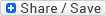27
Wed, Sep

# Alphamatical formula for Attitude

###### Typography
• Smaller Small Medium Big Bigger
• Default Helvetica Segoe Georgia TimesI find this very interesting and meaningful:

IF:
A = 1
B = 2
C = 3
D = 4;
E = 5
F = 6;
G = 7
H = 8;
I = 9
J = 10
K = 11
L = 12
M = 13
N = 14
O = 15
P = 16
Q = 17
R = 18
S = 19
T = 20
U = 21
V = 22
W = 23
X =24
Y = 25
Z = 26.

THEN,

H+A+R+D+W+O+R+K
=8+1+18+4+23+15+18+11
= 98%

K+N+O+W+L+E+D+G+E
=11+14+15+23+12+5+4+7+5
=96%

L+O+V+E
= 12+15+22+5
= 54%

L+U+C+K ;
=12+21+3+
11
= 47%

None of them makes 100%.

Then what makes 100%?

Is it Money?
NO!

M+O+N+E+Y
= 13+15+14+5+25
=72%

NO!

L+E+A+D+E+R+S+H+I+P
=12+5+1+4+5+18+19+8+9+16
=97%

Every problem has a solution, only if we perhaps change our

*ATTITUDE*...

A+T+T+I+T+U+D+E = 1+20+20+9+20+21+4+5 = 100%

It is therefore OUR ATTITUDE towards Life and Work that makes OUR Life 100% Successful.```

With 2021 steering us in the face, what will be your attitude to life and work come 2021?

Amazing Mathematics✅▶️➕⏺️✖️◀️➗⏹️

Via WhatsApp, Author unknown